# RD Sharma Solutions Class 10 Chapter 3 Pair of Linear Equations in Two Variables Exercise 3.7

Here you can get free RD Sharma Solutions for Class 10 Maths Chapter 3 Pair Of Linear Equations In Two Variables Exercise 3.7. All RD Sharma Book Solutions are given here exercise wise for the chapter Pair Of Linear Equations In Two Variables. RD Sharma Solutions are helpful in the preparation of several school level, graduate and undergraduate level competitive exams. Practicing questions from RD Sharma Mathematics Solutions for Class 10 Chapter 3 Pair Of Linear Equations In Two Variables is proven to enhance your math skills.

 Class: Class 10th Chapter: Chapter 3 Exercise: Exercise 3.7 Name: Pair Of Linear Equations In Two Variables

## RD Sharma Solutions Class 10 Chapter 3 Pair Of Linear Equations In Two Variables Exercise 3.7

RD Sharma Class 10 Solutions Chapter 3 for Pair Of Linear Equations In Two Variables Exercise 3.7 are given below.

RD Sharma Solutions Class 10 Chapter 3 Pair Of Linear Equations In Two Variables Exercise 3.7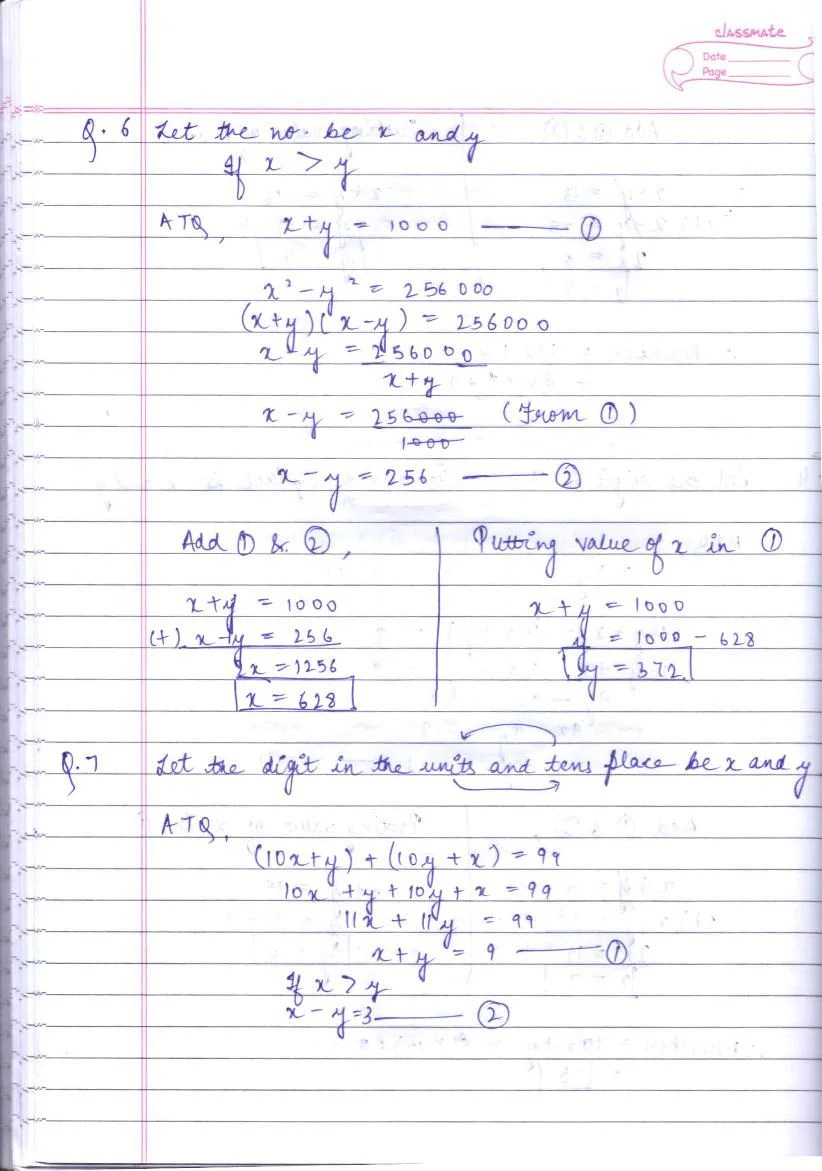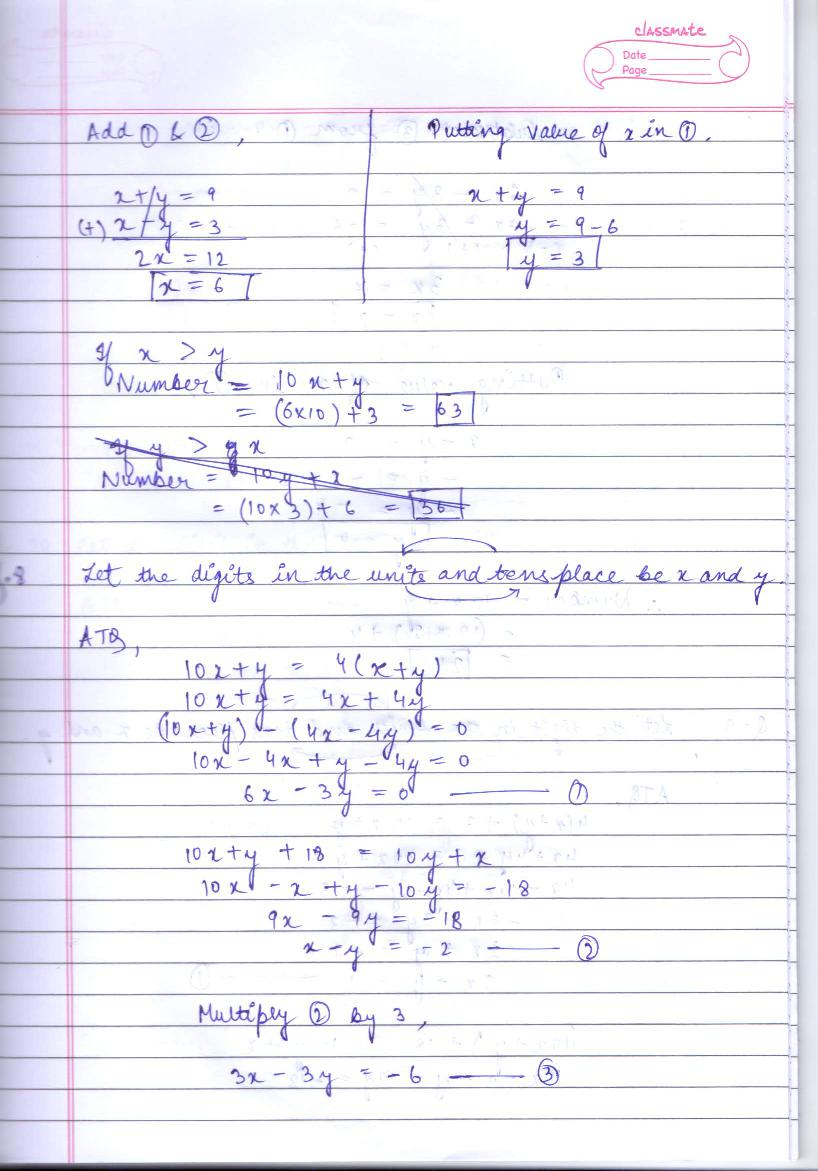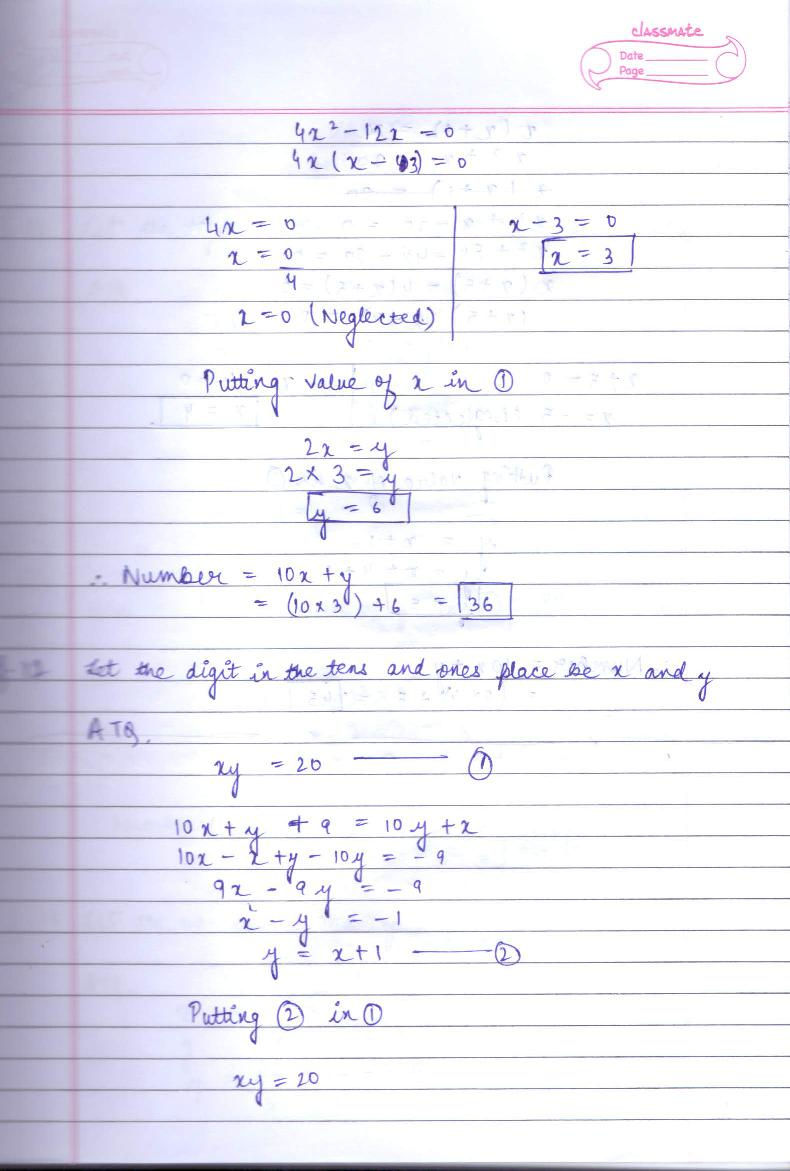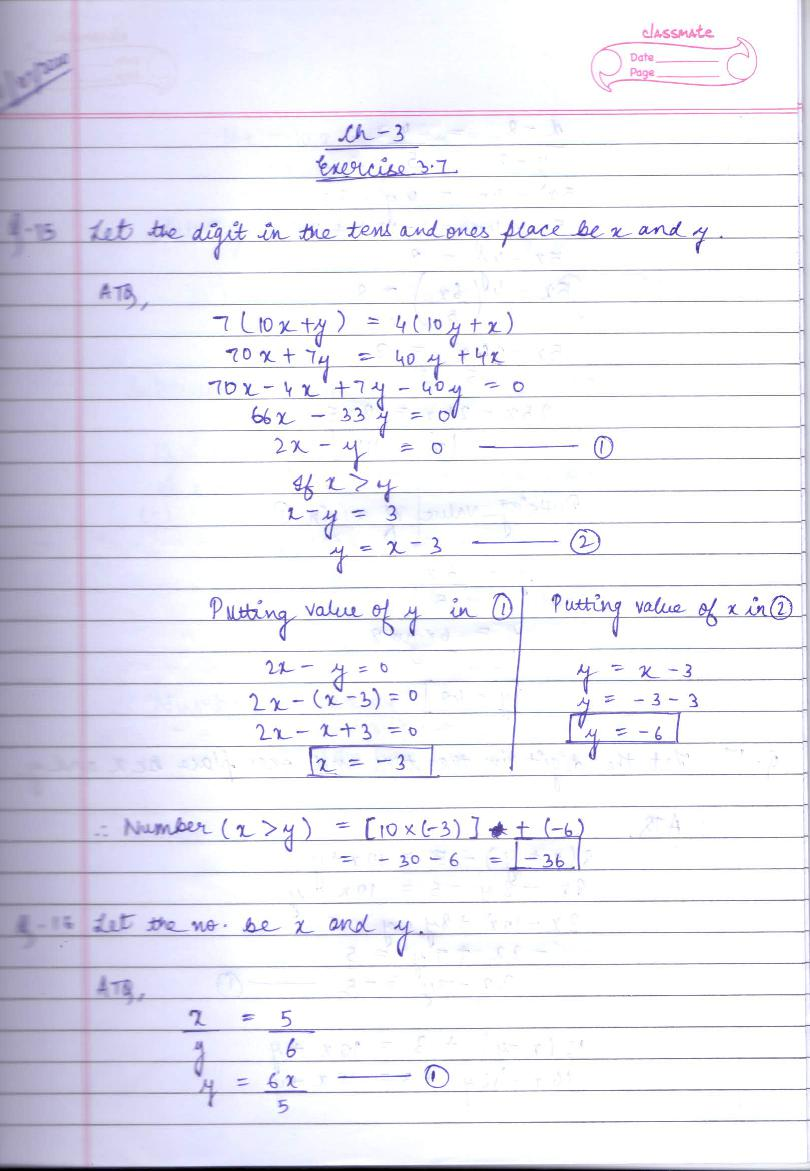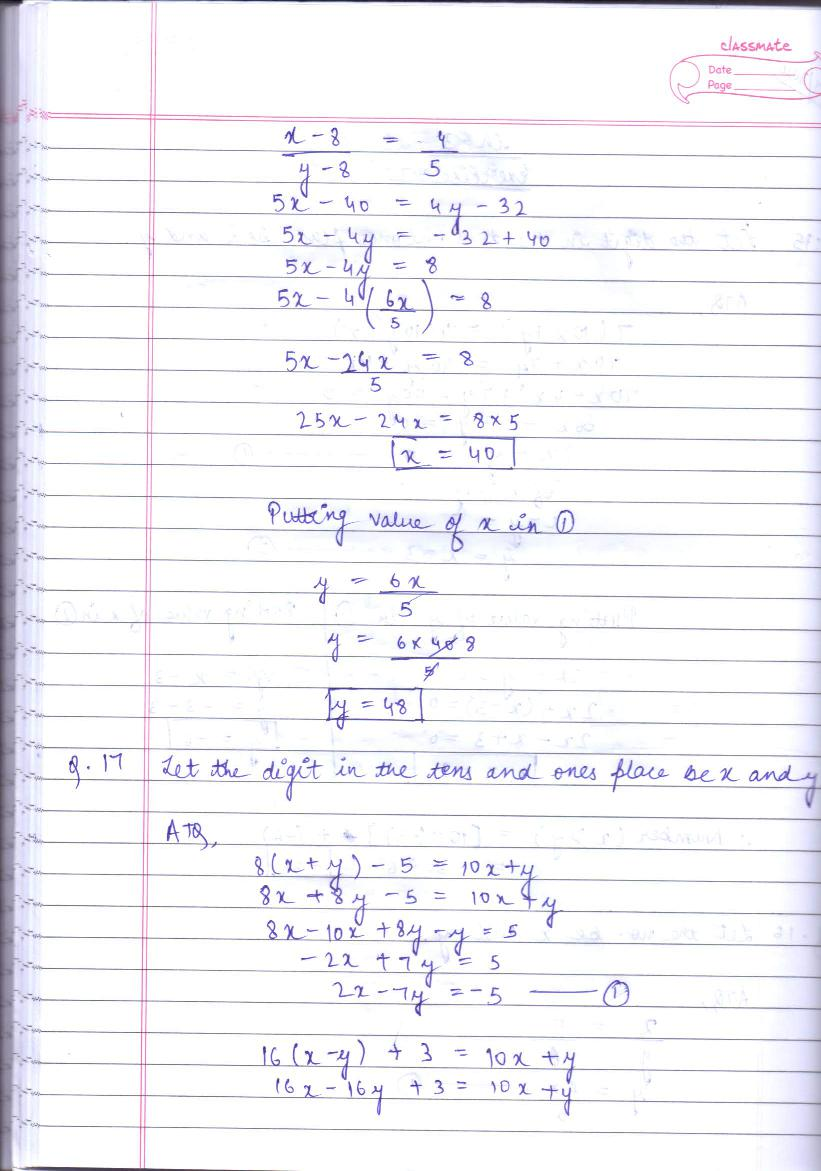RD Sharma Solutions for Class 10 Maths PDF download for Chapter 3 Pair Of Linear Equations In Two Variables Exercise 3.7 is available here for free.

 « Previous Next »

### RD Sharma Solutions Class 10 Chapter 3 Exercise Wise

You can also see RD Sharma Solutions of all exercises of Chapter 3 – Pair Of Linear Equations In Two Variables from here.

### RD Sharma Solutions Class 10 Chapter Wise

If you want RD Sharma solutions of any topic other than Chapter 3 Pair Of Linear Equations In Two Variables, check it from here. Mathematics by RD Sharma has all chapters that are in your Class 10 syllabus.

Click to rate this post!
[Total: 2 Average: 5]